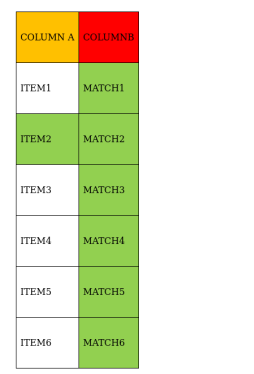# There are 6 items in column A and 6 items in column B.

Question:

There are 6 items in column A and 6 items in column B. A student is asked to match each item in column A with an item in column B. How many possible (correct or incorrect) answers are there to this question?

Solution:As we can see that For Item2 there can be any of the match

So, For each item in column A there are 6 different options in column B since we don't have to think about correct or incorrect matching.

So possible number of combinations possible to answer:

$6 \times 5 \times 4 \times 3 \times 2 \times 1=720$